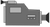# Solutions for Linear Equations using Algebra Tiles

Updated on

Algebra Tiles: Linear Equation Solutions Part 1Algebra Tiles: Linear Equation Solutions Part 2Algebra Tiles: Linear Equation Solutions Part 3Algebra Tiles: Linear Equation Solutions Part 4 (Summary)## 1. Types of Solutions Part 1:

• One unique solution
• The solution could even be 0## 2. Types of Solutions Part 2:

• If after simplifying both sides and no variable is left along with two sides which can never be the same, there is NO SOLUTION!## 3. Types of Solutions Part 3:

If both sides are exactly the same such that simplification yields 0 on both sides, then any value for 'x' is a solution.## 4. Types of Solutions Part 4: Summary